# How to Calculate Debt Service Coverage Ratio

Step-by-Step Solution to Examples

## How to Calculate the Debt Service Coverage Ratio

This guide will describe how to calculate the Debt Service Coverage Ratio. First, we will go over a brief description of the Debt Service Coverage Ratio, why it is important, and then go over step-by-step solutions to several examples of Debt Service Coverage Ratio Calculations.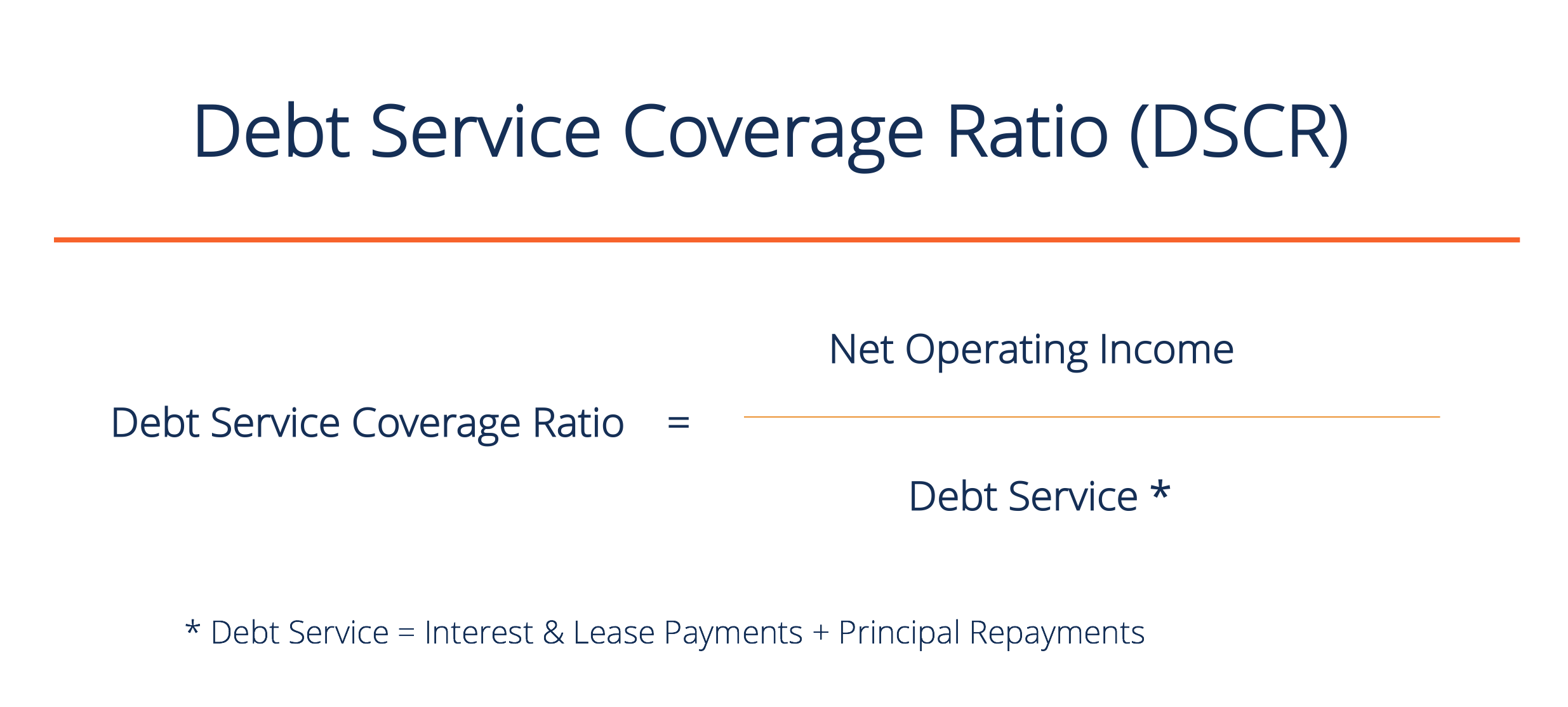### What is the Debt Service Coverage Ratio (DSCR)?

This ratio measures the net operating income available to pay short-term debt. The DSCR is a useful benchmark to measure an individual or firm’s ability to meet their debt payments with cash. A higher ratio implies that the entity is more creditworthy because they have sufficient funds to service their debt obligations – to make the required payments on a timely basis.

### Why is the DSCR important?

The DSCR ratio indicates the financial health of an entity. A lower ratio indicates an increased probability of default or bankruptcy. However, a low ratio does not necessarily mean the company is at risk. A company’s DSCR should be compared to the DSCR of other companies operating in the same industry, and evaluated relative to the industry average. It would be inappropriate to compare an airline company (using large amounts of debt) with a software company (which likely uses more equity financing).

### Example 1 – DSCR Income Statement

In this example, we will calculate the Debt Service Coverage Ratio of Company A. Use the following income statement: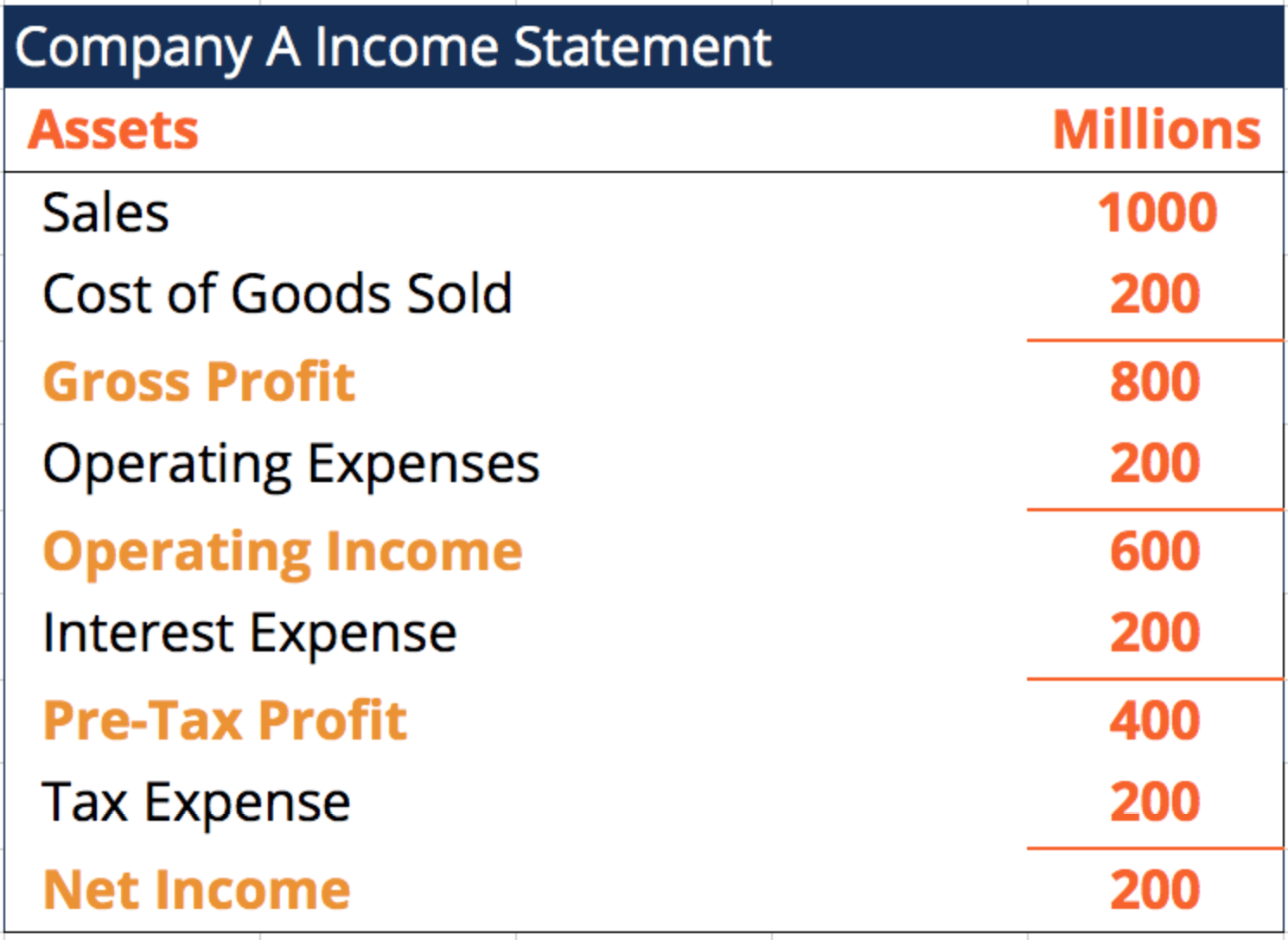Step 1: Write out the formula

DSCR = Net Operating Income / Debt Service

Step 2: Find the Net Operating Income

The operating income is found by subtracting the operating expenses from the firm’s gross profit. In this example, it is equal to \$600M.

Step 3: Find the Debt Service

The debt service will typically be located below the operating income, as the entity must pay their interest and principal payments before tax. Debt service is just the interest expense in this example, which is equal to \$200M.

Step 4: Calculate to find the DSCR

DSCR = Net Operating Income / Debt Service

DSCR = \$600M / \$200M = 3 (or 3x as it’s a ratio)

### Example 2 – DSCR Simple Income Statement

In this example, we will calculate the Debt Service Coverage Ratio of Company B. Use the following income statement: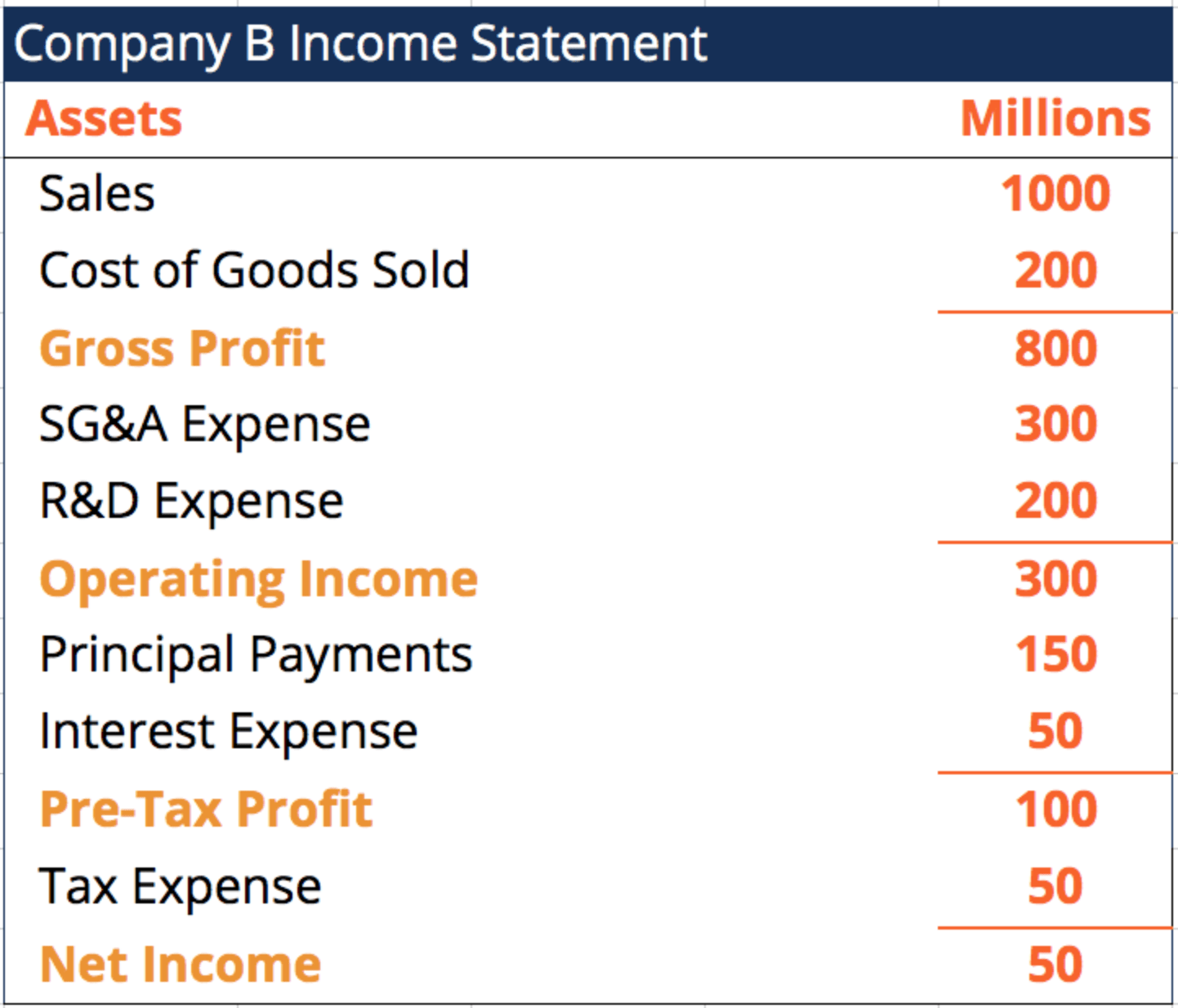Step 1: Write out the formula

DSCR = Net Operating Income / Debt Service

Step 2: Find the Net Operating Income

The operating income is located below the operating expenses (SG&A and R&D expenses). It is equal to \$300M in this example.

Step 3: Find the Debt Service

In this example, the debt service is larger because the firm must pay back principal plus interest payments.

Debt Service = Interest and Lease Payments + Principal Repayment

Debt Service = \$50M + \$150M = \$200M

Step 4: Calculate to find the DSCR

DSCR = Net Operating Income / Debt Service

DSCR = \$300M / \$200M = 1.5 (or 1.5x)

### Example 3 – Missing Elements from Income Statement

In this example, we will calculate the Debt Service Coverage Ratio of Company C. Use the following income statement and information: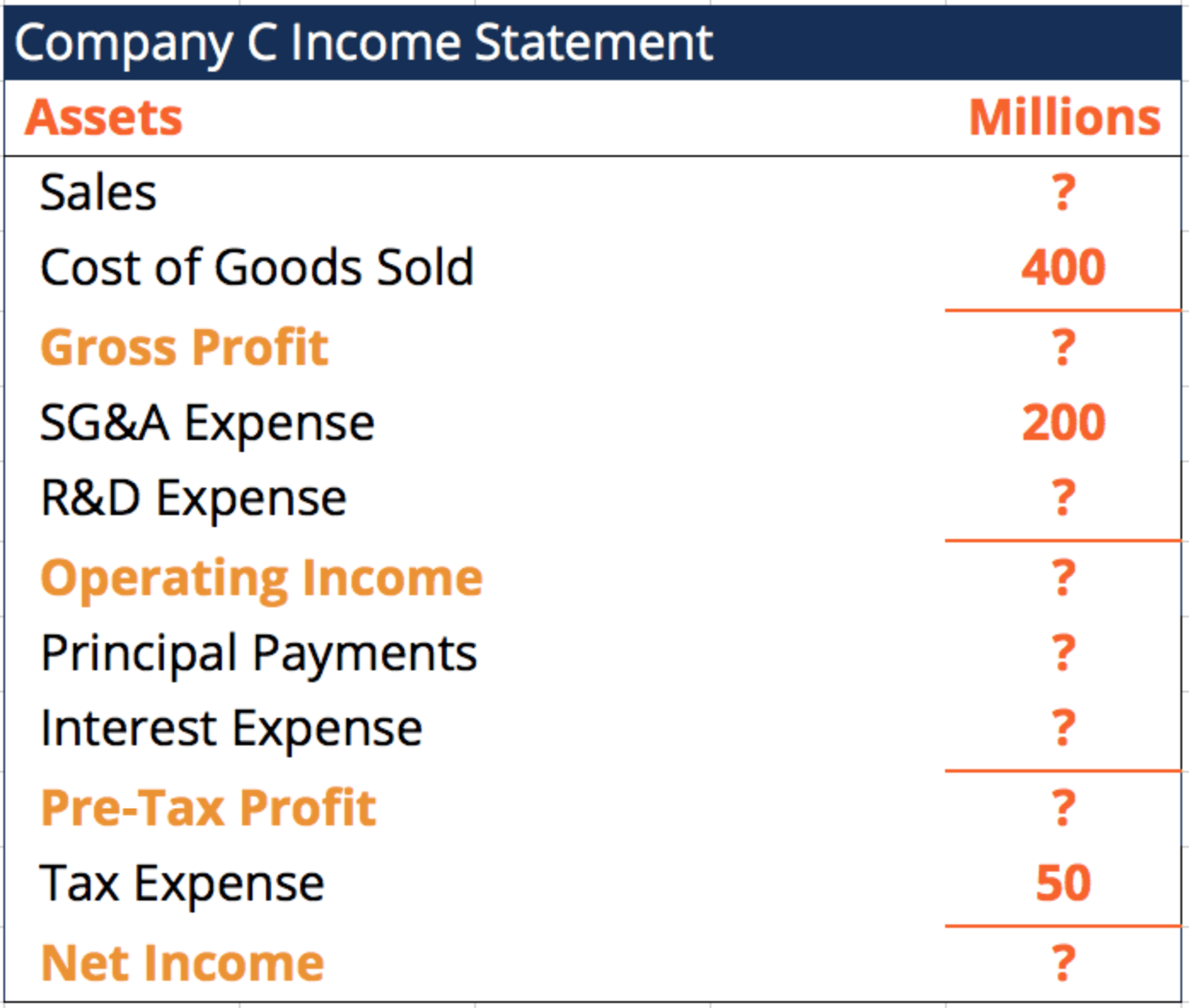Information:

• R&D Expense is 25% of the firm’s SG&A Expense.
• Principal Payments and Interest Expense are each 10% of the firm’s Operating Income.
• Sales are three times the company’s Cost of Goods Sold.

Step 1: Write out the formula

DSCR = Net Operating Income / Debt Service

Step 2: Find the Net Operating Income

To figure out the firm’s Net Operating Income, we must calculate the Sales and R&D Expense (since these values are not provided).

Sales = 3 x Cost of Goods Sold

Sales = 3 x \$400M = \$1200M

R&D Expense = 25% x SG&A Expense

R&D Expense = 25% x \$200M = \$50M

Net Operating Income = Sales – Cost of Goods Sold – SG&A Expense – R&D Expense

Net Operating Income = \$1200M – \$400M – \$200M – \$50M = \$550M

Step 3: Find the Debt Service

Principal Payments and Interest Expense are each 10% of the company’s Operating Income:

Principal Payments = 10% x Operating Income

Principal Payments = 10% x \$550M = \$55M

Interest Expense = 10% x Operating Income

Interest Expense = 10% x \$550M = \$55M

Now find the Debt Service:

Debt Service = Interest & Lease Payments + Principal Repayment

Debt Service = \$55M + \$55M = \$110M

Step 4: Calculate to find the DSCR

DSCR = Net Operating Income / Debt Service

DSCR = \$550M / \$110M = 5 (or 5x)

### Example 4 – DSCR + Fill out Income Statement

In this example, we will calculate the Debt Service Coverage Ratio of Company D. Use the following partial income statement and information: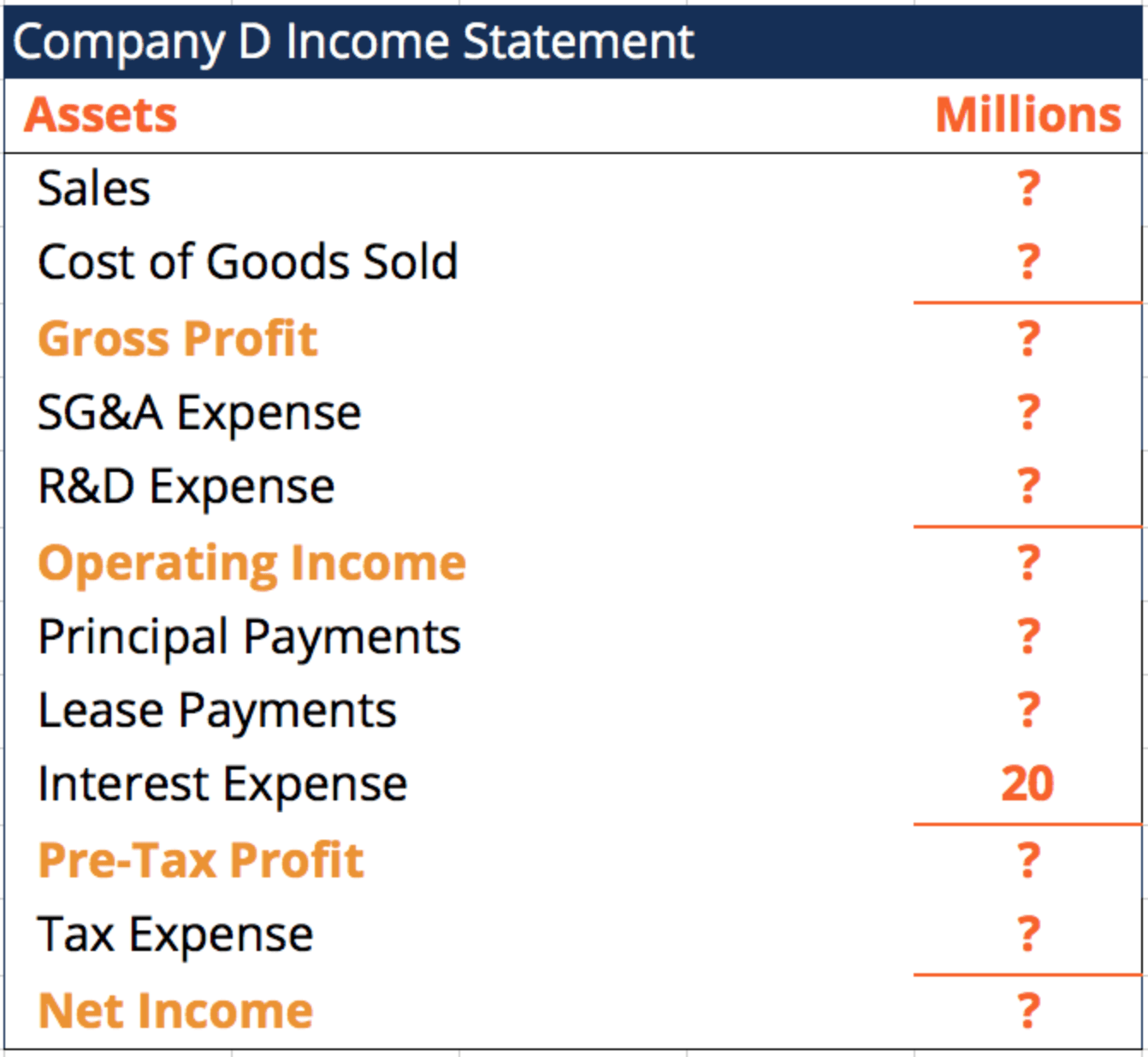Information:

• Tax rate is 50%.
• R&D Expense is \$10M less than half of the firm’s SG&A Expense.
• Principal Payments are double the Pre-Tax Profit.
• Cost of Goods Sold is 60% of Sales.
• Net Income is 25% of the Lease Payments.
• SG&A Expense is 30% of the company’s Sales.
• Lease Payments are double the Interest Expense.

Step 1: Write out the formula

DSCR = Net Operating Income / Debt Service

Step 2: Fill out the income statement

To find the firm’s Net Operating Income, since most line items are blank, we must first fill out the income statement with the information we have:

• Lease Payments

Starting with the Interest Expense of \$20M (the only value we have), we can figure out the Lease Payments since they are double the Interest Expense.

Lease Payments = 2 x \$20M = \$40M

• Net Income

We can now figure out the Net Income (because it is 25% of the Lease Payments).

Net Income = 25% x \$40M = \$10M

• Pre-Tax Profit & Tax Expense

The 50% tax rate implies that the tax expense is 50% of the Pre-Tax Profit. The firm keeps 50% of the Pre-Tax Profit as Net Income. Therefore, we can conclude that the Pre-Tax Profit is double the Net Income.

Pre-Tax Profit = 2 x \$10M = \$20M

Tax Expense = Pre-Tax Profit – Net Income

Tax Expense = \$20M – \$10M = \$10M

• Principal Payments

Principal Payments are double the Pre-Tax Profit

Principal Payments = 2 x Pre-Tax Profit

Principal Payments = 2 x \$20M = \$40M

• Operating Income

Although Operating Income is missing, we can calculate this by adding the line items that we have values for, above the Pre-Tax Profit.

Operating Income = Pre-Tax Profit + Interest Expense + Lease Payments + Principal Payments

Operating Income = \$20M + \$20M + \$40M + \$40M = \$120M

Step 3: Find the Debt Service

Debt Service = Interest & Lease Payments + Principal Repayment

Debt Service = \$20M + \$40M + \$40M = \$100M

Step 4: Calculate to find the DSCR

DSCR = Net Operating Income / Debt Service

DSCR = \$120M / \$100M = 1.2 (or 1.2x)

### Final Word

The Debt Service Coverage Ratio can be a very helpful metric for assessing a company’s overall financial health, and specifically how capable it is of servicing its current debt. The ratio can also assist lenders and investors in determining whether it’s safe for the company to take on additional debt financing. The DSCR should always be looked at relative to the industry average.

The Debt Service Coverage Ratio is an important metric for management and Financial Analysis. To learn more, check out CFI’s Financial Analysis Fundamentals. Here are a few other resources that are related to DSCR:

• Interest Coverage Ratio
• Capital vs Operating Lease
• Profit and Loss Statment (P&L)
• Coverage Ratio

### Financial Analyst Training

Get world-class financial training with CFI’s online certified financial analyst training program!

Gain the confidence you need to move up the ladder in a high powered corporate finance career path.

Learn financial modeling and valuation in Excel the easy way, with step-by-step training.